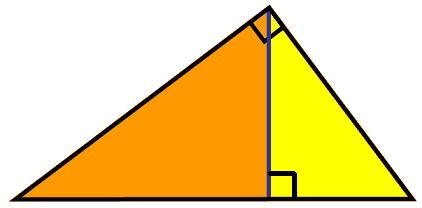#### You may also like### Fitting In

The largest square which fits into a circle is ABCD and EFGH is a square with G and H on the line CD and E and F on the circumference of the circle. Show that AB = 5EF. Similarly the largest equilateral triangle which fits into a circle is LMN and PQR is an equilateral triangle with P and Q on the line LM and R on the circumference of the circle. Show that LM = 3PQ### Look Before You Leap

Can you spot a cunning way to work out the missing length?### Triangle Midpoints

You are only given the three midpoints of the sides of a triangle. How can you construct the original triangle?

# Nicely Similar

##### Age 14 to 16 Challenge Level:Well done to the people who found the height (48) and then used it to get the triangle side lengths, but special congratulations to Michael from Taradale High School for a more direct approach using the powerful property that this arrangement contains three similar triangles.

If the yellow triangle has a hypotenuse, h, and if the yellow-orange triangle and the yellow triangle are similar, then the ratio, h : 100 is equal to the ratio 36 : h

From that we know that h must be 60, and using the same thinking again the hypotenuse of the orange triangle must be 80.